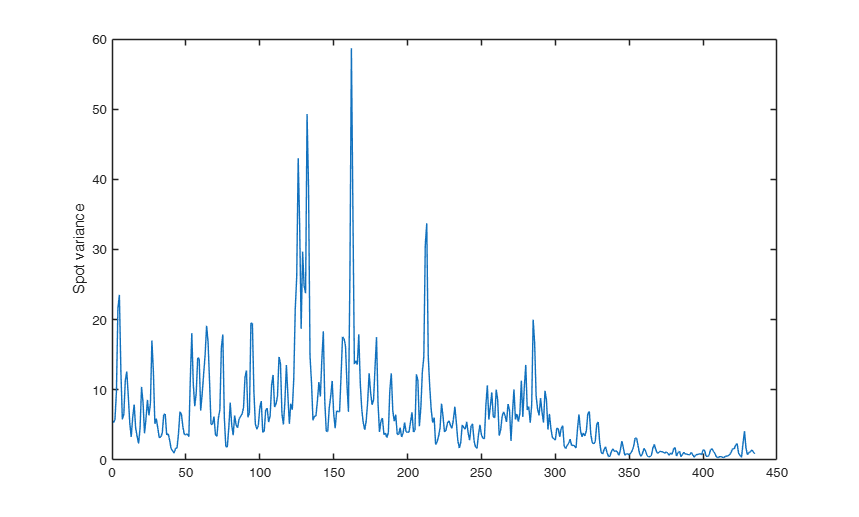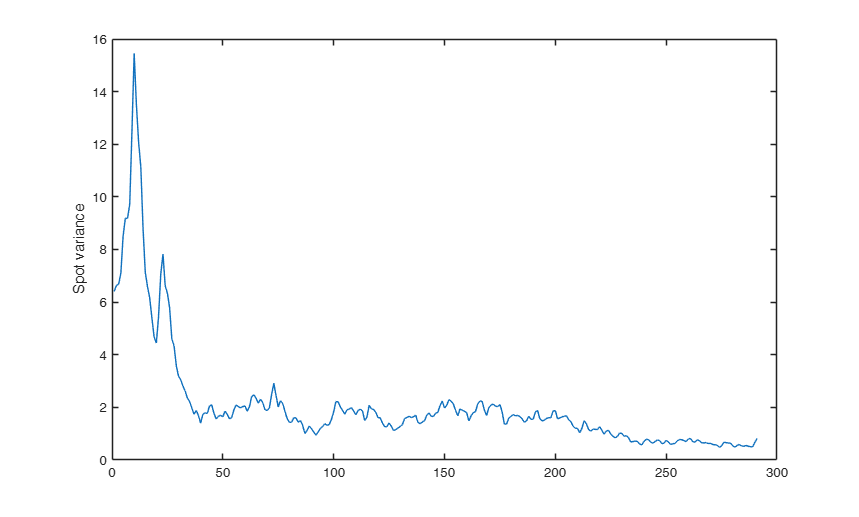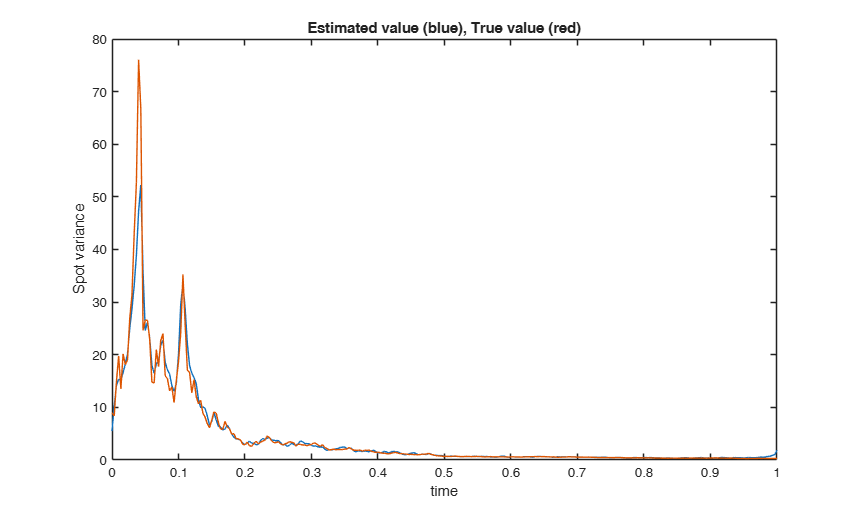# FE_spot_vol_FFT

FE_spot_vol_FFT estimates the instantaneous variance of a diffusion process by the Fourier estimator with Dirichlet kernel, using the FFT algorithm

## Syntax

• spotvar=FE_spot_vol_FFT(x,t)example
• spotvar=FE_spot_vol_FFT(x,t,Name,Value)example
• [spotvar,tau]=FE_spot_vol_FFT(___)example

## Description

 spotvar =FE_spot_vol_FFT(x, t) Example of call of FE_spot_vol_FFT with just two input arguments.

 spotvar =FE_spot_vol_FFT(x, t, Name, Value) FE_spot_vol_FFT called with optional input argument N and M.

 [spotvar, tau] =FE_spot_vol_FFT(___) Example of call of FE_spot_vol_FFT providing both estimation values and times.

## Examples

expand all

###Example of call of FE_spot_vol_FFT with just two input arguments.

Generates price and instantaneous variance from the Constant Elasticity of Variance model [S. Beckers, The Journal of Finance, Vol. 35, No. 3, 1980] and estimates the instantaneous variance via the Fourier method.

n=1000;  T=1; t=0:T/n:T; S0=100;
[S,sigma]=CEVmodel(t,S0); % data generation
x=log(S); % log-price
spotvar=FE_spot_vol_FFT(x,t);
plot(spotvar(2:end-1))
ylabel('Spot variance')###FE_spot_vol_FFT called with optional input argument N and M.

Generates price and instantaneous variance from the Constant Elasticity of Variance model [S. Beckers, The Journal of Finance, Vol. 35, No. 3, 1980] and estimates the instantaneous variance via the Fourier method.

n=21600;  T=1; t=0:T/n:T; S0=100;
[S,sigma]=CEVmodel(t,S0); % data generation
x=log(S); % log-price
N=floor(n/2); M=floor(n^0.5); % cutting frequencies
spotvar=FE_spot_vol_FFT(x,t,'N',N,'M',M);
plot(spotvar(2:end-1))
ylabel('Spot variance')###Example of call of FE_spot_vol_FFT providing both estimation values and times.

Generates price and instantaneous variance from the Constant Elasticity of Variance model [S. Beckers, The Journal of Finance, Vol. 35, No. 3, 1980] and estimates the instantaneous variance via the Fourier method.

n=21600;  T=1; t=0:T/n:T; S0=100;
[S,sigma]=CEVmodel(t,S0); % data generation
x=log(S); % log-price
N=floor(n/2); M=150; % cutting frequencies
[spotvar,tau]=FE_spot_vol_FFT(x,t,'N',N,'M',M);
plot(tau,spotvar)
xlabel('time')
hold on; plot(tau,sigma(1:n/(2*M):end));
ylabel('Spot variance')
title('Estimated value (blue), True value (red)')## Input Arguments

### x — Observation values. Vector.

Row or column vector containing the observed values.

Data Types: single| double

### t — Observation times. Vector.

Row or column vector with the same length of x containing the observation times

Data Types: single| double

### Name-Value Pair Arguments

Specify optional comma-separated pairs of Name,Value arguments. Name is the argument name and Value is the corresponding value. Name must appear inside single quotes (' '). You can specify several name and value pair arguments in any order as  Name1,Value1,...,NameN,ValueN.

Example:  'N',500 , 'M',218 

### N —cutting frequency.scalar.

If N is not specified, it is set equal to (length(x)-1)/2.

Example:  'N',500 

Data Types: single | double

### M —maximum Fourier frequency for spot variance reconstruction.scalar.

If M is not specified, it is set equal to sqrt(length(x))*log(length(x)).

Example:  'M',218 

Data Types: single | double

## Output Arguments

### spotvar —Spot variance.  Vector

Row vector with length 2M+1 of spot variance values at the time grid tau.

### tau —Estimation times.  Vector

Row vector with length 2M+1 where the spot variance is estimated.

## More About

### Additional Details

We assume our timeseries data are discrete observations from a diffusion process $x$ following the It\^o stochastic differential equation $$dx(t)= \sigma(t) \ dW(t) + b(t) \ dt,$$ where $W$ is a Brownian motion on a filtered probability space. Let $\sigma$ and $b$ be random processes, adapted to the Brownian filtration.

See the Reference for further mathematical details.

The squared diffusion coefficient $\sigma^2(t)$ is called instantaneous variance of the process $x$.

For any positive integer $n$, let ${\cal S}_{n}:=\{ 0=t_{0}\leq \cdots \leq t_{n}=T \}$ be the observation times. Moreover, let $\delta_i(x):= x(t_{i+1})-x(t_i)$ be the increments of $x$.

The Fourier estimator of the instantaneous variance for $t \in (0,T)$, is defined as $$\widehat \sigma^2_{n,N,M}(t):= \sum_{|k|\leq M} \left(1- {|k|\over M}\right) e^{{\rm i} {{2\pi}\over {T}} tk} c_k(\sigma^2_{n,N}),$$ where $$c_k(\sigma^2_{n,N}):= {T \over {2N+1}}\sum_{|s|\leq N} c_s(dx_n) c_{k-s}(dx_n),$$ where for any integer $s$, $|s|\leq 2N$, the discretized Fourier coefficients of the increments are $$c_s(dx_{n}):= {1\over {T}} \sum_{i=0}^{n-1} e^{-{\rm i} {{2\pi}\over {T}} st_i}\delta_i(x).$$

Mancino, M.E., Recchioni, M.C., Sanfelici, S. (2017), Fourier-Malliavin Volatility Estimation. Theory and Practice, "Springer Briefs in Quantitative Finance", Springer.

## See Also

This page has been automatically generated by our routine publishFS